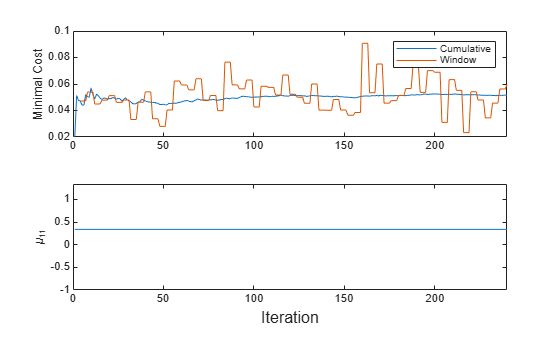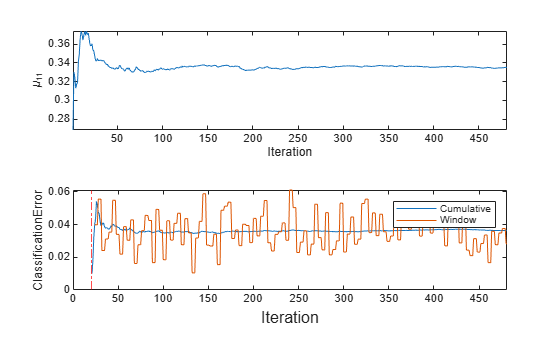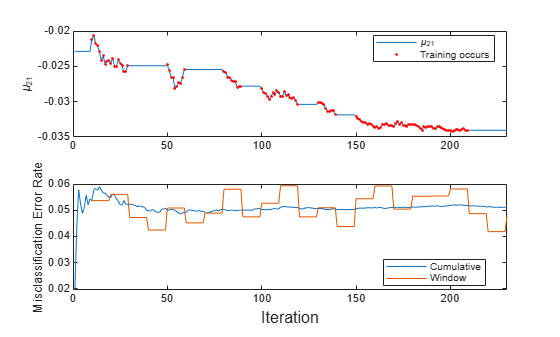Main Content

# updateMetrics

Update performance metrics in naive Bayes classification model for incremental learning given new data

## Syntax

Mdl = updateMetrics(Mdl,X,Y)
Mdl = updateMetrics(Mdl,X,Y,'Weights',Weights)

## Description

Given streaming data, updateMetrics measures the performance of a configured naive Bayes classification model for incremental learning (incrementalClassificationNaiveBayes object). updateMetrics stores the performance metrics in the output model.

updateMetrics allows for flexible incremental learning. After you call the function to update model performance metrics on an incoming chunk of data, you can perform other actions before you train the model to the data. For example, you can decide whether you need to train the model based on its performance on a chunk of data. Alternatively, you can both update model performance metrics and train the model on the data as it arrives, in one call, by using the updateMetricsAndFit function.

To measure the model performance on a specified batch of data, call loss instead.

example

Mdl = updateMetrics(Mdl,X,Y) returns a naive Bayes classification model for incremental learning Mdl, which is the input naive Bayes classification model for incremental learning Mdl modified to contain the model performance metrics on the incoming predictor and response data, X and Y respectively.When the input model is warm (Mdl.IsWarm is true), updateMetrics overwrites previously computed metrics, stored in the Metrics property, with the new values. Otherwise, updateMetrics stores NaN values in Metrics instead.The input and output models have the same data type.

example

Mdl = updateMetrics(Mdl,X,Y,'Weights',Weights) specifies observation weights Weights.

## Examples

collapse all

Train a naive Bayes classification model by using fitcnb, convert it to an incremental learner, and then track its performance to streaming data.

Load and Preprocess Data

Load the human activity data set. Randomly shuffle the data.

load humanactivity rng(1); % For reproducibility n = numel(actid); idx = randsample(n,n); X = feat(idx,:); Y = actid(idx);

For details on the data set, enter Description at the command line.

Train Naive Bayes Classification Model

Fit a naive Bayes classification model to a random sample of half the data.

idxtt = randsample([true false],n,true); TTMdl = fitcnb(X(idxtt,:),Y(idxtt))
TTMdl = ClassificationNaiveBayes ResponseName: 'Y' CategoricalPredictors: [] ClassNames: [1 2 3 4 5] ScoreTransform: 'none' NumObservations: 12053 DistributionNames: {1×60 cell} DistributionParameters: {5×60 cell} Properties, Methods 

TTMdl is a ClassificationNaiveBayes model object representing a traditionally trained model.

Convert Trained Model

Convert the traditionally trained classification model to a naive Bayes classification model for incremental learning.

IncrementalMdl = incrementalLearner(TTMdl)
IncrementalMdl = incrementalClassificationNaiveBayes IsWarm: 1 Metrics: [1×2 table] ClassNames: [1 2 3 4 5] ScoreTransform: 'none' DistributionNames: {1×60 cell} DistributionParameters: {5×60 cell} Properties, Methods 

The incremental model is warm. Therefore, updateMetrics can track model performance metrics given data.

Track Performance Metrics

Track the model performance on the rest of the data by using the updateMetrics function. Simulate a data stream by processing 50 observations at a time. At each iteration:

1. Call updateMetrics to update the cumulative and window minimal cost of the model given the incoming chunk of observations. Overwrite the previous incremental model to update the losses in the Metrics property. Note that the function does not fit the model to the chunk of data—the chunk is "new" data for the model.

2. Store the minimal cost and mean of the first predictor in the first class ${\mu }_{11}$.

% Preallocation idxil = ~idxtt; nil = sum(idxil); numObsPerChunk = 50; nchunk = floor(nil/numObsPerChunk); mc = array2table(zeros(nchunk,2),'VariableNames',["Cumulative" "Window"]); mu11 = [IncrementalMdl.DistributionParameters{1,1}(1); zeros(nchunk,1)]; Xil = X(idxil,:); Yil = Y(idxil); % Incremental fitting for j = 1:nchunk ibegin = min(nil,numObsPerChunk*(j-1) + 1); iend = min(nil,numObsPerChunk*j); idx = ibegin:iend; IncrementalMdl = updateMetrics(IncrementalMdl,Xil(idx,:),Yil(idx)); mc{j,:} = IncrementalMdl.Metrics{"MinimalCost",:}; mu11(j + 1) = IncrementalMdl.DistributionParameters{1,1}(1); end

IncrementalMdl is an incrementalClassificationNaiveBayes model object that has tracked the model performance to observations in the data stream.

Plot a trace plot of the performance metrics and estimated coefficient ${\mu }_{11}$.

figure; subplot(2,1,1) h = plot(mc.Variables); xlim([0 nchunk]); ylabel('Minimal Cost') legend(h,mc.Properties.VariableNames) subplot(2,1,2) plot(mu11) ylabel('\mu_{11}') xlim([0 nchunk]); xlabel('Iteration')The cumulative loss is stable, whereas the window loss jumps.

${\mu }_{11}$ does not change because updateMetrics does not fit the model to the data.

Create a naive Bayes classification model for incremental learning by calling incrementalClassificationNaiveBayes and specifying a maximum of 5 expected classes in the data. Specify tracking misclassification error rate in addition to minimal cost.

Mdl = incrementalClassificationNaiveBayes('MaxNumClasses',5,'Metrics',"classiferror");

Mdl is an incrementalClassificationNaiveBayes model. All its properties are read-only.

Determine whether the model is warm and the size of the metrics warm-up period by querying model properties.

isWarm = Mdl.IsWarm
isWarm = logical 0 
mwp = Mdl.MetricsWarmupPeriod
mwp = 1000 

Mdl.IsWarm is 0; therefore, Mdl is not warm.

Determine the number of observations incremental fitting functions, such as fit, must process before measuring the performance of the model.

numObsBeforeMetrics = Mdl.MetricsWarmupPeriod
numObsBeforeMetrics = 1000 

Load the human activity data set. Randomly shuffle the data.

load humanactivity n = numel(actid); rng(1) % For reproducibility idx = randsample(n,n); X = feat(idx,:); Y = actid(idx);

For details on the data set, enter Description at the command line.

Suppose that the data collected when the subject was not moving (Y <= 2) has double the quality than when the subject was moving. Create a weight variable that attributes 2 to observations collected from a still subject, and 1 to a moving subject.

W = ones(n,1) + ~Y;

Implement incremental learning by performing the following actions at each iteration:

• Simulate a data stream by processing a chunk of 50 observations.

• Measure model performance metrics on the incoming chunk using updateMetrics. Specify the corresponding observation weights and overwrite the input model.

• Fit the model to the incoming chunk. Specify the corresponding observation weights and overwrite the input model.

• Store ${\mu }_{11}$ and the misclassification error rate to see how they evolve during incremental learning.

% Preallocation numObsPerChunk = 50; nchunk = floor(n/numObsPerChunk); ce = array2table(zeros(nchunk,2),'VariableNames',["Cumulative" "Window"]); mu11 = zeros(nchunk,1); % Incremental learning for j = 1:nchunk ibegin = min(n,numObsPerChunk*(j-1) + 1); iend = min(n,numObsPerChunk*j); idx = ibegin:iend; Mdl = updateMetrics(Mdl,X(idx,:),Y(idx),'Weights',W(idx)); ce{j,:} = Mdl.Metrics{"ClassificationError",:}; Mdl = fit(Mdl,X(idx,:),Y(idx),'Weights',W(idx)); mu11(j) = Mdl.DistributionParameters{1,1}(1); end

IncrementalMdl is an incrementalClassificationNaiveBayes model object trained on all the data in the stream.

To see how the parameters evolved during incremental learning, plot them on separate subplots.

figure; subplot(2,1,1) plot(mu11) ylabel('\mu_{11}') xlabel('Iteration') axis tight subplot(2,1,2) plot(ce.Variables); ylabel('ClassificationError') xline(numObsBeforeMetrics/numObsPerChunk,'r-.'); xlabel('Iteration') xlim([0 nchunk]); legend(ce.Properties.VariableNames)mdlIsWarm = numObsBeforeMetrics/numObsPerChunk
mdlIsWarm = 20 

The plot suggests that fit always fits the model to the data, and updateMetrics does not track the classification error until after the metrics warm-up period (20 chunks).

Incrementally train a naive Bayes classification model only when its performance degrades.

Load the human activity data set. Randomly shuffle the data.

load humanactivity n = numel(actid); rng(1) % For reproducibility idx = randsample(n,n); X = feat(idx,:); Y = actid(idx);

For details on the data set, enter Description at the command line.

Configure a naive Bayes classification model for incremental learning so that the maximum number of expected classes is 5, the tracked performance metric includes the misclassification error rate, and the metrics window size of 1000. Fit the configured model to the first 1000 observations.

Mdl = incrementalClassificationNaiveBayes('MaxNumClasses',5,'MetricsWindowSize',1000,... 'Metrics','classiferror'); initobs = 1000; Mdl = fit(Mdl,X(1:initobs,:),Y(1:initobs));

Mdl is an incrementalClassificationNaiveBayes model object.

Perform incremental learning, with conditional fitting, by following this procedure for each iteration:

• Simulate a data stream by processing a chunk of 100 observations at a time.

• Update the model performance on the incoming chunk of data.

• Fit the model to the chunk of data only when the misclassification error rate is greater than 0.05.

• When tracking performance and fitting, overwrite the previous incremental model.

• Store the misclassification error rate and the mean of the first predictor in the second class ${\mu }_{21}$ to see how they evolve during training.

• Track when fit trains the model.

% Preallocation numObsPerChunk = 100; nchunk = floor((n - initobs)/numObsPerChunk); mu21 = zeros(nchunk,1); ce = array2table(nan(nchunk,2),'VariableNames',["Cumulative" "Window"]); trained = false(nchunk,1); % Incremental fitting for j = 1:nchunk ibegin = min(n,numObsPerChunk*(j-1) + 1 + initobs); iend = min(n,numObsPerChunk*j + initobs); idx = ibegin:iend; Mdl = updateMetrics(Mdl,X(idx,:),Y(idx)); ce{j,:} = Mdl.Metrics{"ClassificationError",:}; if ce{j,2} > 0.05 Mdl = fit(Mdl,X(idx,:),Y(idx)); trained(j) = true; end mu21(j) = Mdl.DistributionParameters{2,1}(1); end

Mdl is an incrementalClassificationNaiveBayes model object trained on all the data in the stream.

To see how the model performance and ${\mu }_{21}$ evolved during training, plot them on separate subplots.

subplot(2,1,1) plot(mu21) hold on plot(find(trained),mu21(trained),'r.') ylabel('\mu_{21}') legend('\mu_{21}','Training occurs','Location','best') hold off subplot(2,1,2) plot(ce.Variables) ylabel('Misclassification Error Rate') xlabel('Iteration') legend(ce.Properties.VariableNames,'Location','best')The trace plot of ${\mu }_{21}$ shows periods of constant values, during which the loss within the previous 1000 observation window is at most 0.05.

## Input Arguments

collapse all

Incremental learning model whose performance is measured, specified as an incrementalClassificationNaiveBayes model object. You can create Mdl directly or by converting a supported, traditionally trained machine learning model using the incrementalLearner function. For more details, see the corresponding reference page.

If Mdl.IsWarm is false, updateMetrics does not track the performance of the model. Before updateMetrics can track performance metrics, you must perform all the following actions:

Chunk of predictor data with which to measure the model performance

The length of the observation labels Y and the number of observations in X must be equal; Y(j) is the label of observation j (row or column) in X.

Note

• If Mdl.NumPredictors = 0, updateMetrics infers the number of predictors from X, and sets the congruent property of the output model. Otherwise, if the number of predictor variables in the streaming data changes from Mdl.NumPredictors, updateMetrics issues an error.

• updateMetrics supports only floating-point input predictor data. If the input model Mdl represents a converted, traditionally trained model fit to categorical data, use dummyvar to convert each categorical variable to a numeric matrix of dummy variables, and concatenate all dummy variable matrices and any other numeric predictors. For more details, see Dummy Variables.

Data Types: single | double

Chunk of labels with which to measure the model performance, specified as a categorical, character, or string array, logical or floating-point vector, or cell array of character vectors.

The length of the observation labels Y and the number of observations in X must be equal; Y(j) is the label of observation j (row or column) in X. updateMetrics issues an error when at least one of the conditions is met:

• Y contains a newly encountered label and the maximum number of classes has been reached previously (see MaxNumClasses and ClassNames arguments of incrementalClassificationNaiveBayes).

• The data types of Y and Mdl.ClassNames are different.

Data Types: char | string | cell | categorical | logical | single | double

Chunk of observation weights, specified as a floating-point vector of positive values. updateMetrics weighs the observations in X with the corresponding values in Weights. The size of Weights must equal n, which is the number of observations in X.

By default, Weights is ones(n,1).

For more details, including normalization schemes, see Observation Weights.

Data Types: double | single

Note

If an observation (predictor or label) or weight Weight contains at least one missing (NaN) value, updateMetrics ignores the observation. Consequently, updateMetrics uses fewer than n observations to compute the model performance.

## Output Arguments

collapse all

Updated naive Bayes classification model for incremental learning, returned as an incremental learning model object of the same data type as the input model Mdl, an incrementalClassificationNaiveBayes object.

If the model is not warm, updateMetrics does not compute performance metrics. As a result, the Metrics property of Mdl remains completely composed of NaN values. If the model is warm, updateMetrics computes the cumulative and window performance metrics on the new data X and Y, and overwrites the corresponding elements of Mdl.Metrics. All other properties of the input model Mdl carry over to the output model Mdl. For more details, see Performance Metrics.

## Tips

• Unlike traditional training, incremental learning might not have a separate test (holdout) set. Therefore, to treat each incoming chunk of data as a test set, pass the incremental model and each incoming chunk to updateMetrics before training the model on the same data using fit.

## Algorithms

collapse all

### Performance Metrics

• updateMetrics tracks only model performance metrics, specified by the row labels of the table in Mdl.Metrics, from new data when the incremental model is warm (IsWarm property is true). An incremental model is warm when the fit function performs both of the following actions:

• Fit the incremental model to Mdl.MetricsWarmupPeriod observations, which is the metrics warm-up period.

• Fit the incremental model to all expected classes (see MaxNumClasses and ClassNames arguments of incrementalClassificationNaiveBayes)

• Mdl.Metrics stores two forms of each performance metric as variables (columns) of a table, Cumulative and Window, with individual metrics in rows. When the incremental model is warm, updateMetrics updates the metrics at the following frequencies:

• Cumulative — The function computes cumulative metrics since the start of model performance tracking. The function updates metrics every time you call it and bases the calculation on the entire supplied data set.

• Window — The function computes metrics based on all observations within a window determined by the Mdl.MetricsWindowSize property. Mdl.MetricsWindowSize also determines the frequency at which the software updates Window metrics. For example, if Mdl.MetricsWindowSize is 20, the function computes metrics based on the last 20 observations in the supplied data (X((end – 20 + 1):end,:) and Y((end – 20 + 1):end)).

Incremental functions that track performance metrics within a window use the following process:

1. For each specified metric, store a buffer of length Mdl.MetricsWindowSize and a buffer of observation weights.

2. Populate elements of the metrics buffer with the model performance based on batches of incoming observations, and store corresponding observations weights in the weights buffer.

3. When the buffer is filled, overwrite Mdl.Metrics.Window with the weighted average performance in the metrics window. If the buffer is overfilled when the function processes a batch of observations, the latest incoming Mdl.MetricsWindowSize observations enter the buffer, and the earliest observations are removed from the buffer. For example, suppose Mdl.MetricsWindowSize is 20, the metrics buffer has 10 values from a previously processed batch, and 15 values are incoming. To compose the length 20 window, the function uses the measurements from the 15 incoming observations and the latest 5 measurements from the previous batch.

### Observation Weights

For each conditional predictor distribution, updateMetrics computes the weighted average and standard deviation.

If the prior class probability distribution is known (in other words, the prior distribution is not empirical), updateMetrics normalizes observation weights to sum to the prior class probabilities in the respective classes. This action implies that the default observation weights are the respective prior class probabilities.

If the prior class probability distribution is empirical, the software normalizes the specified observation weights to sum to 1 each time you call updateMetrics.

## See Also

### Functions

Introduced in R2021a

Download ebook